# 圆与旋转矩形的碰撞检测（下篇）

2015/02/16 10:28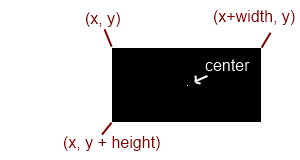x’= cos(theta) * (cx – originX) – sin(theta) * (cy – originY) + originX

y’= sin(theta) * (cx – originX) + cos(theta) * (cy – originY) + originY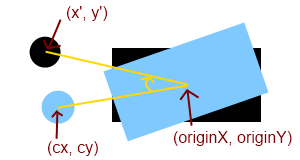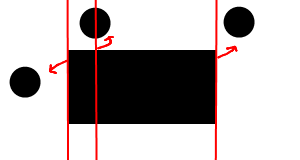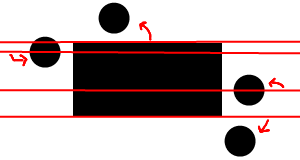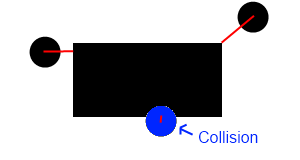1 2 3 4 5 6 7 8 9 10 11 // Rotate circle's center point backdouble unrotatedCircleX = Math.cos(rect.angle) * (circle.x - rect.centerX) -  Math. sin (rect.angle) * (circle.y - rect.centerY) + rect.centerX; double  unrotatedCircleY  = Math. sin (rect.angle) * (circle.x - rect.centerX) +  Math. cos (rect.angle) * (circle.y - rect.centerY) + rect.centerY;  // Closest point in the rectangle to the center of circle rotated backwards(unrotated)double closestX, closestY; // Find the unrotated closest x point from center of unrotated circleif (unrotatedCircleX  < rect.x) closestX = rect.x; else  if  (unrotatedCircleX  > rect.x + rect.width) closestX = rect.x + rect.width; else closestX = unrotatedCircleX ;  // Find the unrotated closest y point from center of unrotated circleif (unrotatedCircleY < rect.y) closestY = rect.y; else  if  (unrotatedCircleY > rect.y + rect.height) closestY = rect.y + rect.height; else closestY = unrotatedCircleY;  // Determine collisionboolean collision = false; double distance = findDistance(unrotatedCircleX , unrotatedCircleY, closestX, closestY);if (distance < circle.radius) collision =  true ;  // Collisionelse collision =  false ;
 1 2 3 4 5 6 7 8 9 /**   * Pythagorean theorem   * @param fromX   * @param fromY   * @param toX   * @param toY   */ public  double  findDistance( double  fromX,  double  fromY,  double  toX,  double  toY){   double  a = Math. abs (fromX - toX);   double  b = Math. abs (fromY - toY);      return  Math. sqrt ((a * a) + (b * b));}### 作者的其它热门文章

0
0 收藏

0 评论
0 收藏
0